# NCERT Solutions for Class 6 Maths Chapter 4 Basic Geometrical Ideas Ex 4.1

NCERT Solutions for Class 6 Maths Chapter 4 Basic Geometrical Ideas Ex 4.1 are part of NCERT Solutions for Class 6 Maths. Here we have given NCERT Solutions for Class 6 Maths Chapter 4 Basic Geometrical Ideas Ex 4.1. https://mcqquestions.guru/ncert-solutions-for-class-6-maths-chapter-4/

 Board CBSE Textbook NCERT Class Class 6 Subject Maths Chapter Chapter 4 Chapter Name Basic Geometrical Ideas Exercise Ex 4.1 Number of Questions Solved 6 Category NCERT Solutions

## NCERT Solutions for Class 6 Maths Chapter 4 Basic Geometrical Ideas Ex 4.1

Question 1.
Use the figure to name:
(a) Five points
(b) A line
(c) Four rays
(d) Five line segments.
Solution :
(a) O, B, C. D, E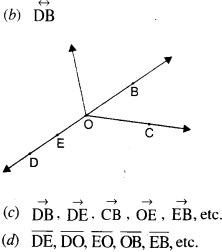Question 2.
Name the line given in all possible (twelve) ways, choosing only two letters at a time from the four given.
Solution :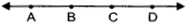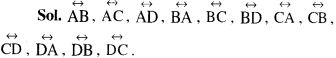Question 3.
Use the figure to name:
(a) The line containing point E.
(b) The line passing through A.
(c) The line on which O lies
(d) Two pairs of intersecting lines.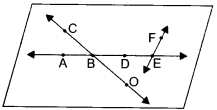Solution :
(a) $$\overleftrightarrow { AE }$$, etc.
(b) $$\overleftrightarrow { AE }$$, etc.
(c) $$\overleftrightarrow { CO } or\overleftrightarrow { OC }$$
(d) $$\overleftrightarrow { CO, } \overleftrightarrow { AE } ;\overleftrightarrow { AE } ,\overleftrightarrow { EF }.$$

Question 4.
How many lines can pass through
(a) one given point?
(b) two given points?
Solution :
(a) Countless lines can pass through one given point.
(b) One and only one line can pass through two given points.

Question 5.
Draw a rough figure and label suitably in each of the following cases :
(a) Point P lies on $$\bar { AB }$$
(b) $$\overleftrightarrow { XY }$$ and $$\overleftrightarrow { PQ }$$ intersect at M.
(c) Line contains E and F but not D.
(d) $$\bar { Op }$$ and $$\bar { OQ }$$ meet at O.
Solution :
(a)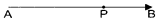(b)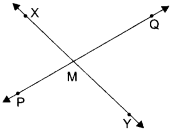(c)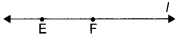(d)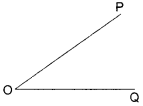Question 6.
Consider the following figure of line $$\bar { MN }$$ Say whether following statements are true or false in context of the given figure.
(a) Q, M, O, N, P are points on the line $$\bar { MN }$$
(b) M, O, N are points on a line segment $$\bar { MN }$$.
(c) M and N are end points of line segment $$\bar { MN }$$.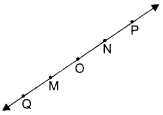(d) O and N are end points of line segment $$\bar { OP }$$.
(e) M is one of the end points of line segment $$\bar { QO }$$.
(f) M is point on ray $$\overrightarrow { OP }$$.
(g) Ray $$\overrightarrow { OP }$$ is different from ray $$\overrightarrow { QP }$$.
(h) Ray $$\overrightarrow { OP }$$ is same as ray $$\overrightarrow { OM }$$.
(i) Ray $$\overrightarrow { OM }$$ is not opposite to ray $$\overrightarrow { OP }$$.
(j) O is not an initial point of $$\overrightarrow { OP }$$.
(k) N is the initial point of $$\overrightarrow { NP }$$ and $$\overrightarrow { NM }$$.
Solution :
(a) True
(b) True
(c) True
(d) False
(e) False
(f) False
(g) True
(h) False
(i) False
(j) False
(k) True.

We hope the NCERT Solutions for Class 6 Maths Chapter 4 Basic Geometrical Ideas Ex 4.1 help you. If you have any query regarding NCERT Solutions for Class 6 Maths Chapter 4 Basic Geometrical Ideas Ex 4.1, drop a comment below and we will get back to you at the earliest.